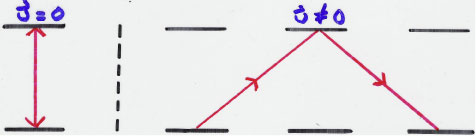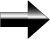Take a moment to make sure you understand this scenario.  The distance that the light travels is longer in the situation on the right.  Therefore, since distance equals rate times time, either the rate (the speed of light) or the time the light traveled for must be different in the right than the left since the distances are different.

When all of the scientists of the day where saying it was time that was constant Einstein was saying that it was not time that was constant, but the speed of light, c.  This meant that the flow of time was different for each person.  This was a revolutionary idea.Special Relativity